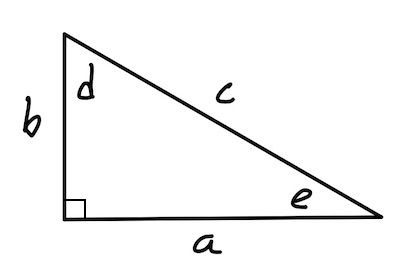## Lesson goal: Solving a right triangle

Previous: Coloring a map | Home | Next: Simulating digital circuits (1)

Suppose your job was to solve the right triangle shown below, meaning find every side and every angle:To do so, you'd have to know at something about at least some sides and/or some angles from the start. Wouldn't it be neat if we could tell the computer what we know about the triangle and have it tell us how to find the rest? Let's code some Prolog do so this.

Some immediate questions arise: 1) how do we represent the knowledge of math and trig. in code? 2) How would we code a way of using this knowledge to explain to us how to solve the triangle? It turns out that Prolog is well suited to handle both of these issues.

We won't go so far as to try and have the computer 'discover' math on its own. We'll tell it the relationships that hold for a right triangle, and let it do what it wants with the relationships. There are two parts, 1) the trigonometry and 2) the mathematics.

• As for the trig: If we look at the triangle, we see 8 laws that hold: 1) $c^2=a^2+b^2$, 2) $d+e+90=180$, and two each for $\sin$, $\cos$, and $\tan$, of both angles $d$ and $e$.

• As for the math: If an equation has $n$ variables in it, if we know $n-1$ of the variables, we can use the equation to find the missing variable. This missing variable, once found, can be thought as a "new knowledge" we can make available to future equations.
Let's focus on the representing the trig knowledge first. We'll define a predicate called rule that works as shown here:
rule([var1,var2,...],text-description)

You can see the 8 rule statements in the code below.
• rule([c,a,b],'c^2=a^2+b^2') means "if you know any two of c, a, or b, you can use 'c^2=a^2+b^2' to find the third."

• rule([d,e],'d+e+90=180') means "if you know either variable d or e, you can use 'd+e+90=180' to solve for the other."

• rule([d,a,c],'sin(d)=a/c') means "if you know any two of d, a, or c, you can use 'sin(d)=a/c' to find the third."
You can likely figure the rest out for yourself.f